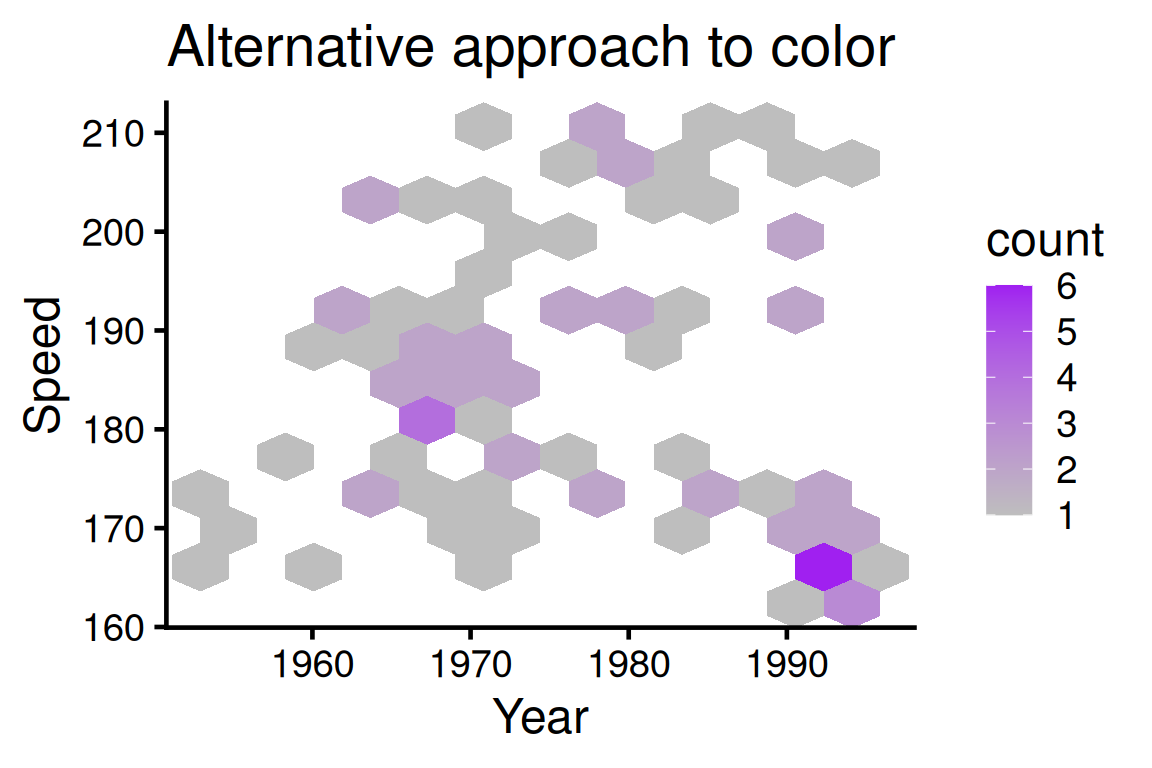# Chapter 11 Two continuous variables

In this chapter, we will look at techniques that explore the relationships between two continuous variables.

## 11.1 Scatterplot

### 11.1.1 Basics and implications

For the following example, we use data set `SpeedSki`.

``````library(GDAdata)
library(ggplot2)

ggplot(SpeedSki, aes(Year, Speed)) +
geom_point() +
labs(x = "Birth year", y = "Speed achieved (km/hr)") +
ggtitle("Skiers by birth year and speed achieved")``````In our example, we simply use `geom_point` on variables `Year` and `Speed` to create the scatterplot. we try to capture if there is a relationship between the age of a player and the speed he/she can achieve. From the graph, it seems such relationship does not exist. Overall, scatterplots are very useful in understanding the correlation (or lack thereof) between variables. The scatterplot gives a good idea of whether that relationship is positive or negative and if there’s a correlation. However, don’t mistake correlation in a scatterplot for causation!

### 11.1.2 Overplotting

In some situations a scatter plot faces the problem of overplotting as there are so many points overlapping. Consider the following example from class. To save time, we randomly sample 20% of the data in advance.

``````library(dplyr)
library(ggplot2movies)

sample <- slice_sample(movies,prop=0.2)

geom_point() +
theme_classic() +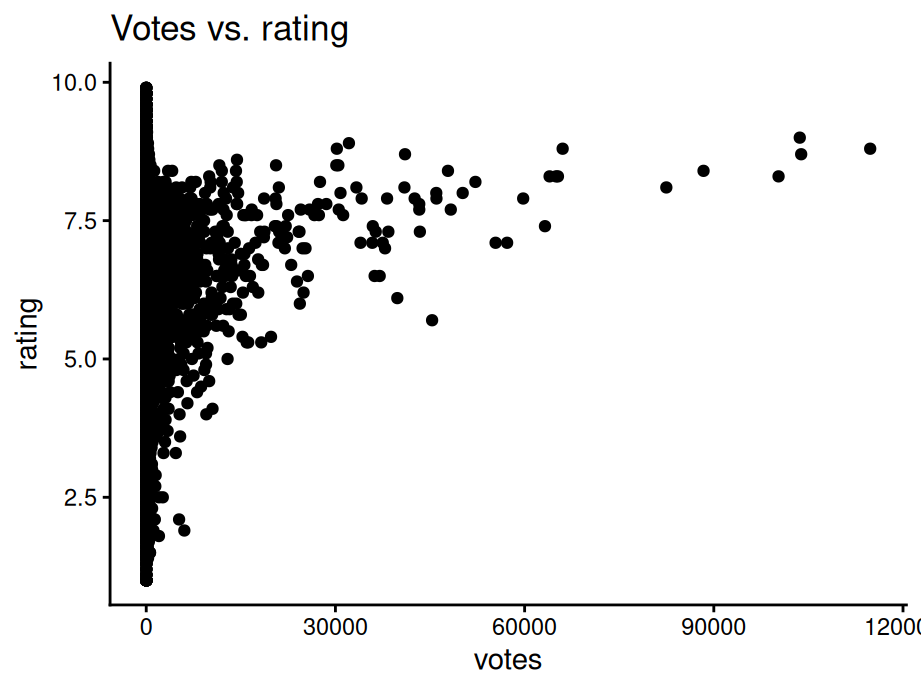To create better visuals, we can use:

• Alpha blending - `alpha=...`

• Open circles - `pch=21`

• smaller circles - `size=...` or `shape="."`

``````library(gridExtra)

geom_point(alpha=0.3) +
theme_classic() +
ggtitle("Alpha blending")

geom_point(pch = 21) +
theme_classic() +
ggtitle("Open circle")

geom_point(size=0.5) +
theme_classic() +
ggtitle("Smaller circle")

grid.arrange(f1, f2, f3,nrow = 3)``````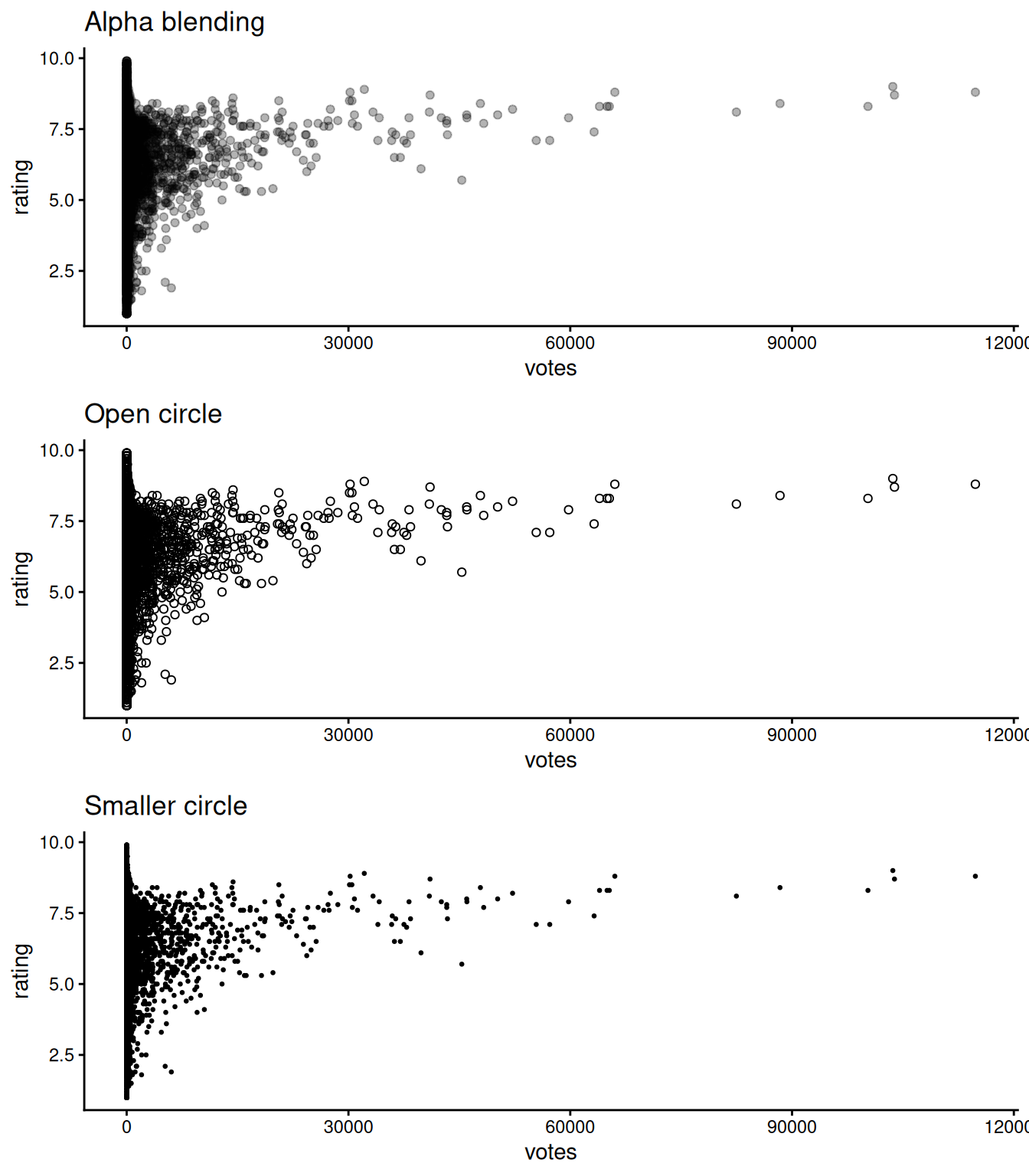Other methods that directly deal with the data:

• Randomly sample data - as shown in the first code chunk using `sample_n`

• Subset - split data into bins using `ntile(votes, 10)`

• Remove outliers

• Transform to log scale

### 11.1.3 Interactive scatterplot

You can create an interactive scatterplot using `plotly`. In the following example, we take 1% of the movie data set to present a better visual. We plotted the votes vs. rating and grouped by the year they are released. In this graph:

• You can hover on to the points to see the title of the movie

• You can double click on the year legend to look at a certain year

• You can zoom into a certain part of the graph to better understand the data points.

``````library(plotly)

sample2 <- slice_sample(movies,prop=0.01) %>%
filter(year > 2000)

plot_ly(sample2, x = ~votes, y = ~rating,
color = ~as.factor(year), text= ~title,
hoverinfo = 'text') ``````

### 11.1.4 Modifications

#### 11.1.4.1 Contour lines

Contour lines give a sense of the density of the data at a glance.

For these contour maps, we will use the `SpeedSki` dataset.

Contour lines can be added to the plot using geom_density_2d() and contour lines work best when combined with other layers

``````ggplot(SpeedSki, aes(Year, Speed)) +
geom_density_2d(bins=5) +
geom_point() +
ggtitle("Scatter plot with contour line")``````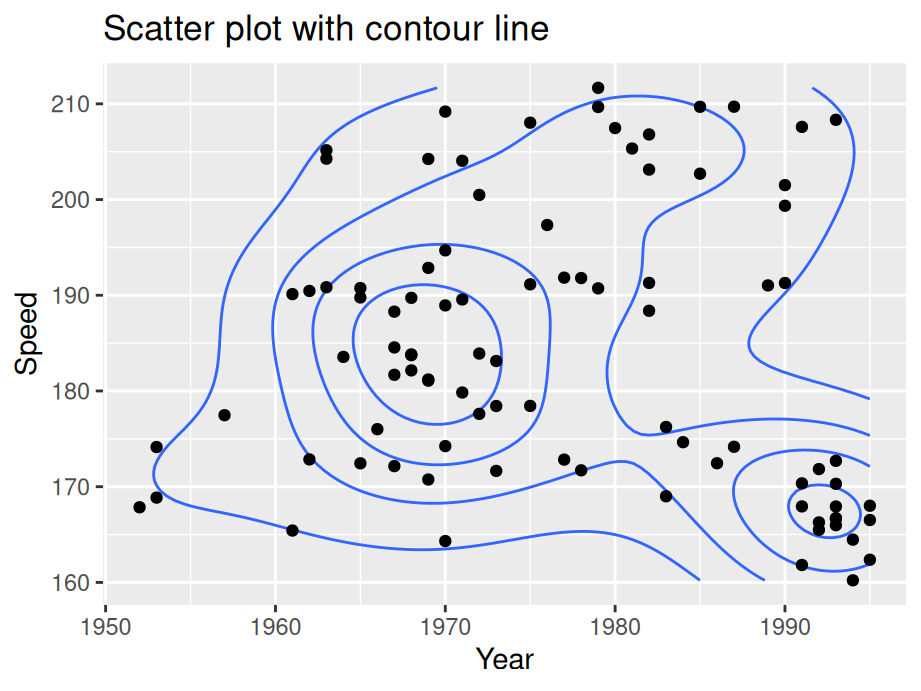You can use `bins` to control the number of contour bins.

#### 11.1.4.2 Scatterplot matrices

If you want to compare multiple parameters to each other, consider using a scatterplot matrix. This will allow you to show many comparisons in a compact and efficient manner.

For these scatterplot matrices, we use the `movies` dataset from the `ggplot2movies` package.

As a default, the base R plot() function will create a scatterplot matrix when given multiple variables:

``````sample3 <- slice_sample(movies,prop=0.01) #sample data

splomvar <- sample3 %>%

plot(splomvar)``````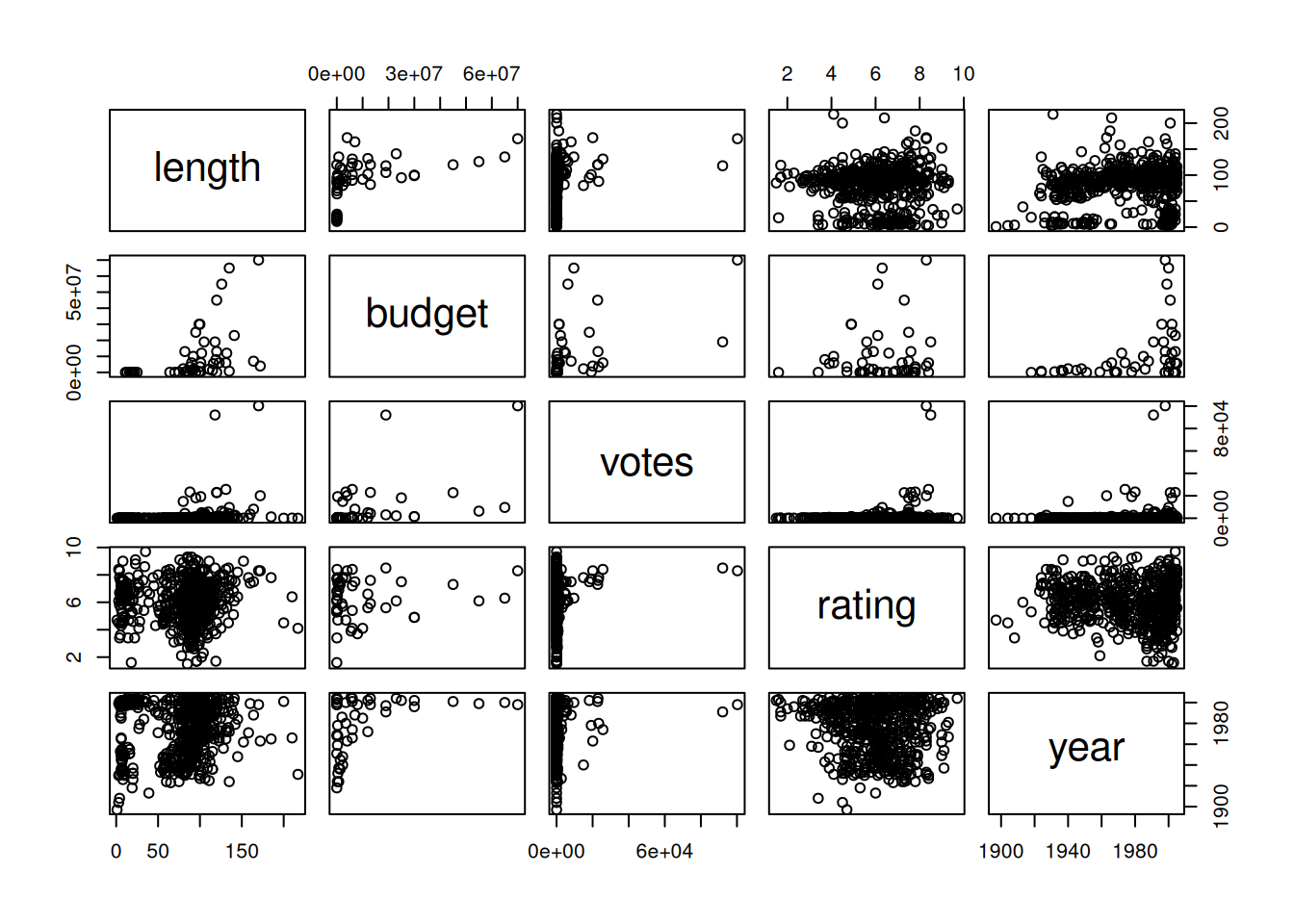While this is quite useful for personal exploration of a datset, it is not recommended for presentation purposes. Something called the Hermann grid illusion makes this plot very difficult to examine.

## 11.2 Heatmaps

### 11.2.1 Basics and implications

In the following example, we still use the `SpeedSki` data set.

``````ggplot(SpeedSki, aes(Year, Speed)) +
geom_bin2d() ``````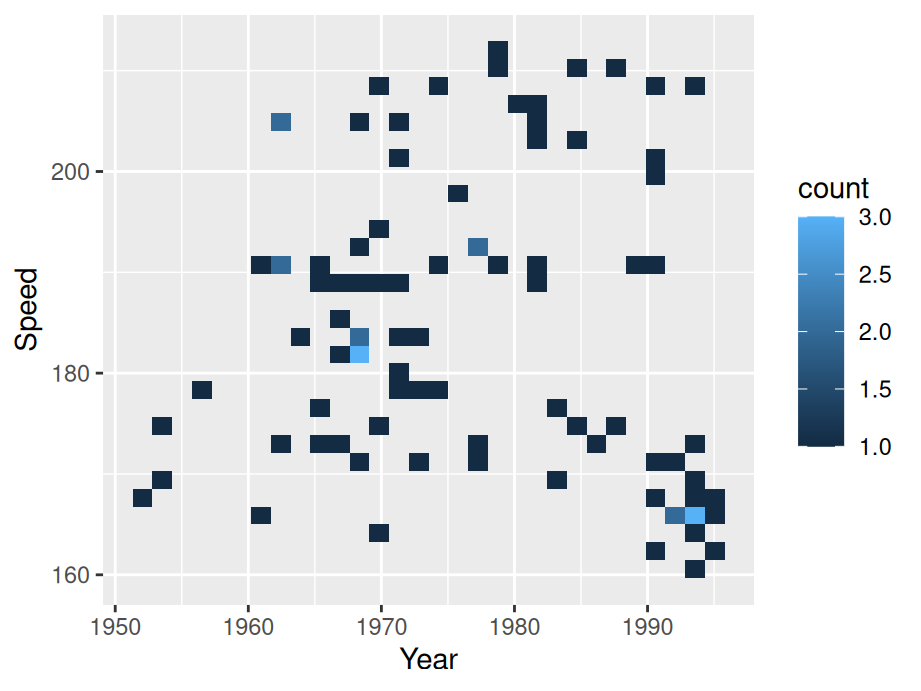To create a heatmap, simply substitute `geom_point()` with `geom_bin2d()`. Generally, heat maps are like a combination of scatterplots and histograms: they allow you to compare different parameters while also seeing their relative distributions.

### 11.2.2 Modifications

For the following section, we introduce some variations on heatmaps.

#### 11.2.2.1 Change number of bins / binwidth

By default, `geom_bin2d()` use 30 bins. Similar to a histogram, we can change the number of bins or binwidth.

``````ggplot(SpeedSki, aes(Year, Speed)) +
geom_bin2d(binwidth = c(5,5)) +
ggtitle("Changing binwidth")``````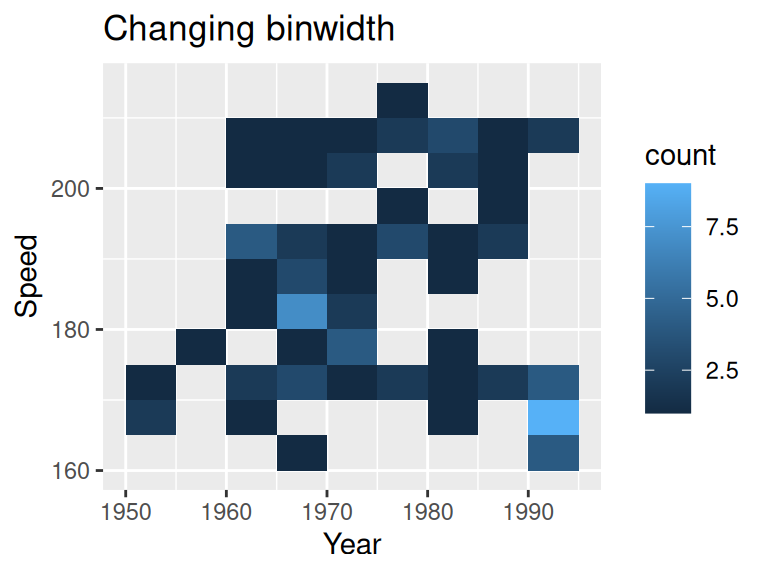Notice we are specifying the binwidth for both x and y axis.

#### 11.2.2.2 Combine with a scatterplot

``````ggplot(SpeedSki, aes(Year, Speed)) +
geom_bin2d(binwidth = c(10, 10), alpha = .4) +
geom_point(size = 2) +
ggtitle("Combined with scatterplot")``````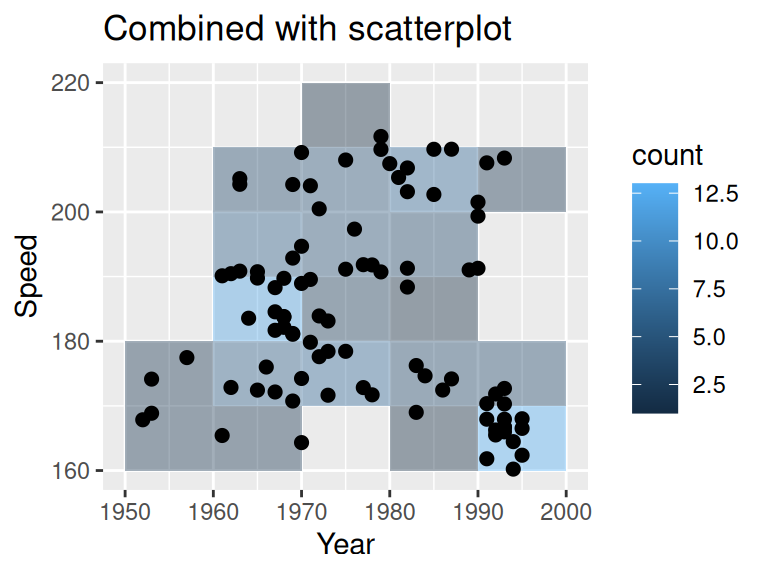#### 11.2.2.3 Change color scale

You can change the continuous scale of color

``````ggplot(SpeedSki, aes(Year, Speed)) +
geom_bin2d() +
ggtitle("Changing color scale") +
scale_fill_viridis_c()``````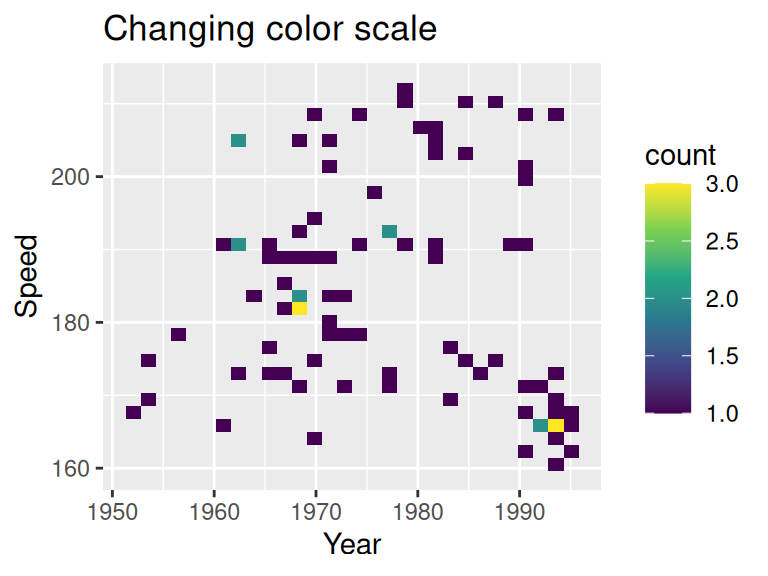#### 11.2.2.4 Hex heatmap

One alternative is a hex heatmap. You can create the graph using `geom_hex`

``````ggplot(SpeedSki, aes(Year, Speed)) +
geom_hex(binswidth = c(10,10)) +
ggtitle("Hex heatmap")``````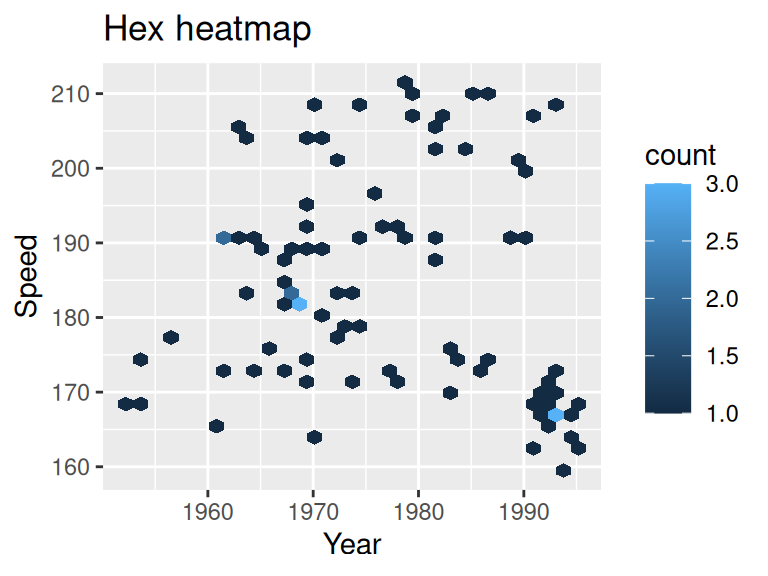#### 11.2.2.5 Alternative approach to color

If you look at all the previous examples, you might notice that lighter points corresponds to more clustered points, which is somewhat counter-intuitive. The following exmaple suggest an alternative approach in color scale.

``````ggplot(SpeedSki, aes(Year, Speed)) +
geom_hex(bins=12) +
scale_fill_gradient(low = "grey", high = "purple") +
theme_classic(18) +
ggtitle("Alternative approach to color")``````